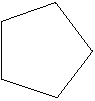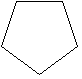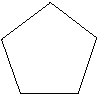Skill 20D Congruence In geometry, the word "congruence" means identical in shape and size. A figure can still be congruent however if it is rotated somewhat. If you have to explain the word congruence to your student you should practice this skill. Look at the figure below the number 1. Which figure is congruent to this figure? 1. A. B. C.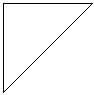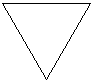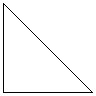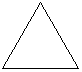Look at the figure below the number 2. Which figure is congruent to this figure? 2. A. B. C.